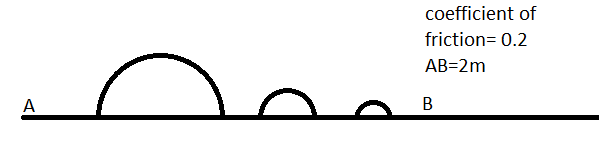# Find net work doneA particle of mass 'm' is moved from point A to B along the track as as shown in the figure

Find the minimum work that has to be done to cause that

DETAILS AND ASSUMPTION

$m=1 kg$

$g=10m/s^2$.

$AB= 2m$

coefficient of friction = $0.2$

Height of first hemisphere = $0.2m$

Height of 2nd hemisphere= $0.1 m$

Height of 3rd hemisphere= $0.01m$

×